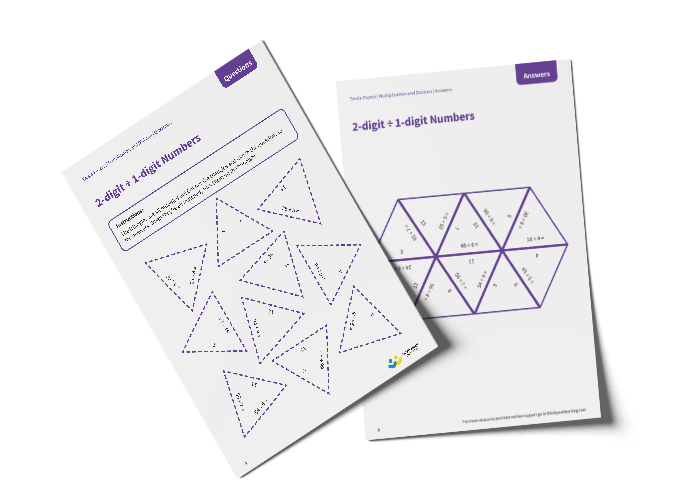# Tarsia Puzzle 2-digit Divide by 1-digit Numbers (Year 3)

The triangles in the tarsia puzzle have all been mixed up. In this 2-digit divided by 1-digit numbers tarsia puzzle, pupils will need to match the division question to the correct answer to create one large hexagon.

This Year 3 2-digit divide by 1-digit numbers tarsia puzzle worksheet contains:

• One question sheet
• One template sheet

Year 3

Division